# Box and Whisker Plots Lesson Plan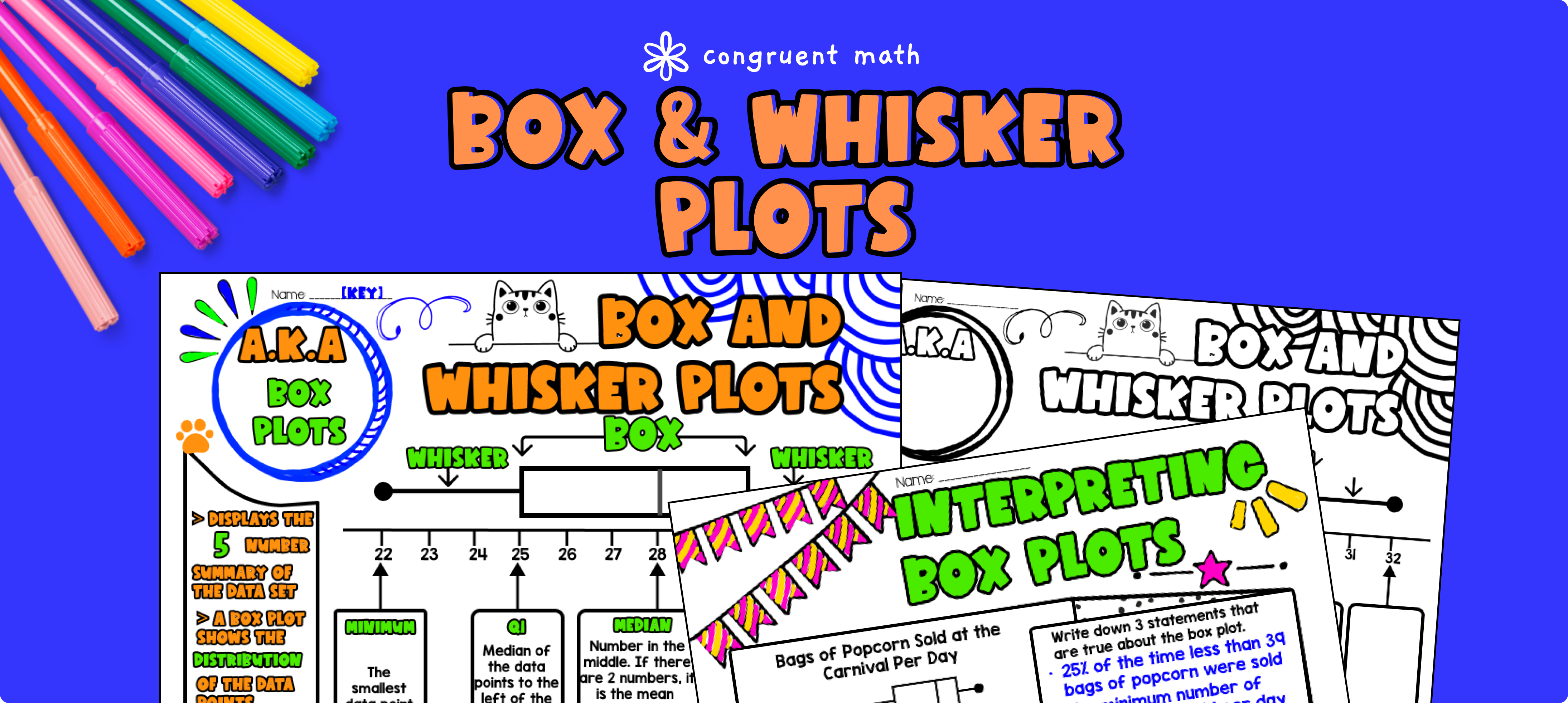Pin This

## Overview

Ever wondered how to teach box and whisker plots, a.k.a. box plots, in an engaging way to your 6th grade students?

In this lesson plan, students will learn about steps to constructing box and whisker plots, interpreting data from box plots, and their real-life applications. Through artistic, interactive guided notes, check for understanding, maze, doodle & color by number activity, students will gain a comprehensive understanding of box and whisker plots.

The lesson culminates with a real-life example that explores how circles are used in amusement park rides like roller coasters and ferris wheels.

## Get the Lesson Materials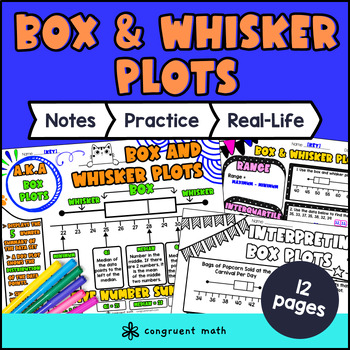\$4.25

## Learning Objectives

After this lesson, students will be able to:

• Construct and interpret box and whisker plots

• Interpret box and whisker plots

• Identify real-life applications of box and whisker plots

## Prerequisites

Before this lesson, students should be familiar with:

• How to calculate median (click here for lesson on mean, median, mode)

• Understanding of how to put numbers in order from least to greatest

## Key Vocabulary

• Box plot

• Box and whiskers plots

• Minimum

• Maximum

• Lower Quartile

• Upper Quartile

• Median

• Interquartile range (IQR)

• Range

• Outlier

## Procedure

### Introduction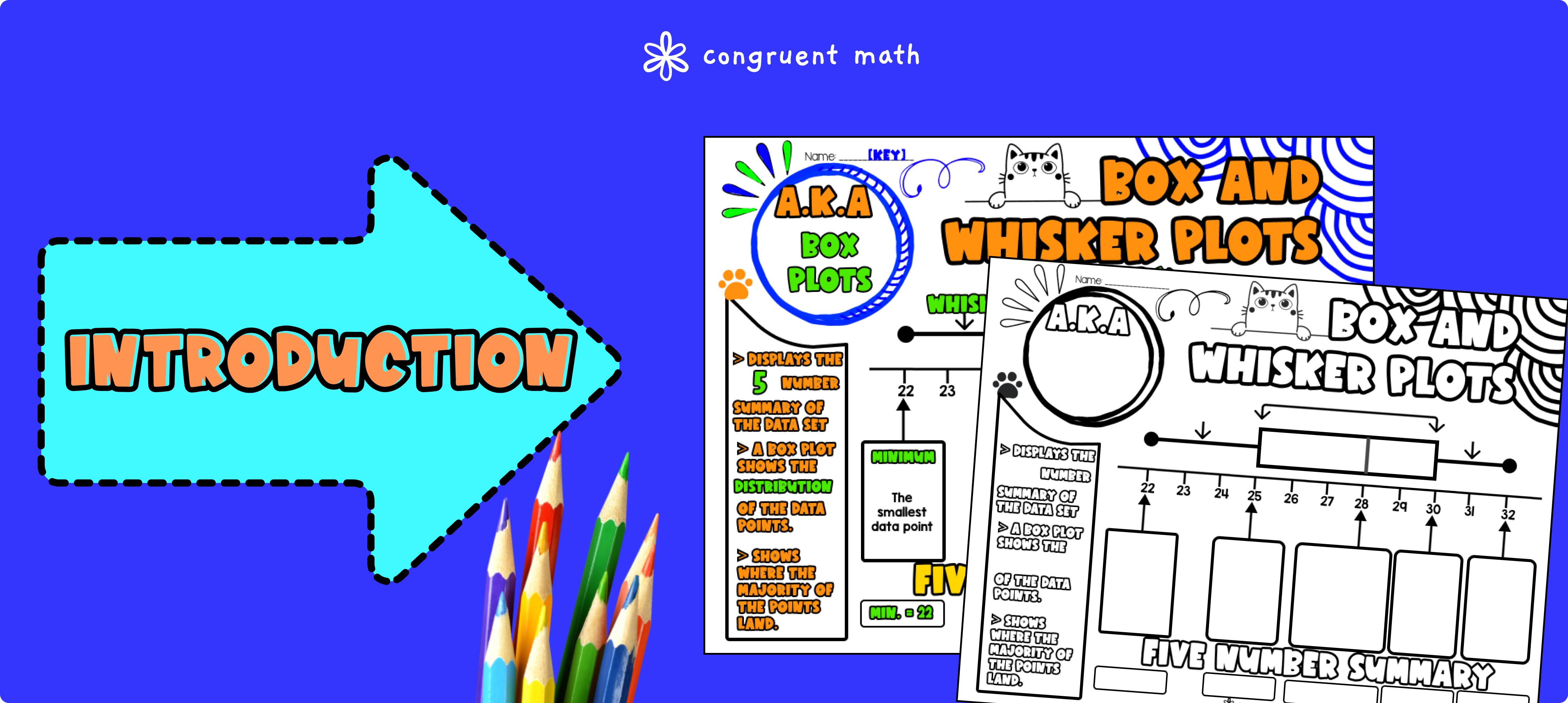Pin This

As a hook, ask students why understanding data visualization is important in real life. It may be helpful to refer to the last page of the guided notes as well as the FAQs below for ideas.

Use the guided notes to introduce the concept of box and whisker plots. Walk through the key points of the steps to construct a box and whisker plots. Review minimum, maximum, median, upper quartile, and lower quartile. Refer to the FAQ below for a walk through on this, as well as ideas on how to respond to common student questions.

Then, walk though the steps of interpreting box and whisker plots, including interquartile range and range.

There are practice problems integrated in the guided notes. You can do the first example together as a class. Then, call on students to talk through their answers, potentially on the whiteboard or projector. Based on student responses, reteach concepts that students need extra help with.

### Practice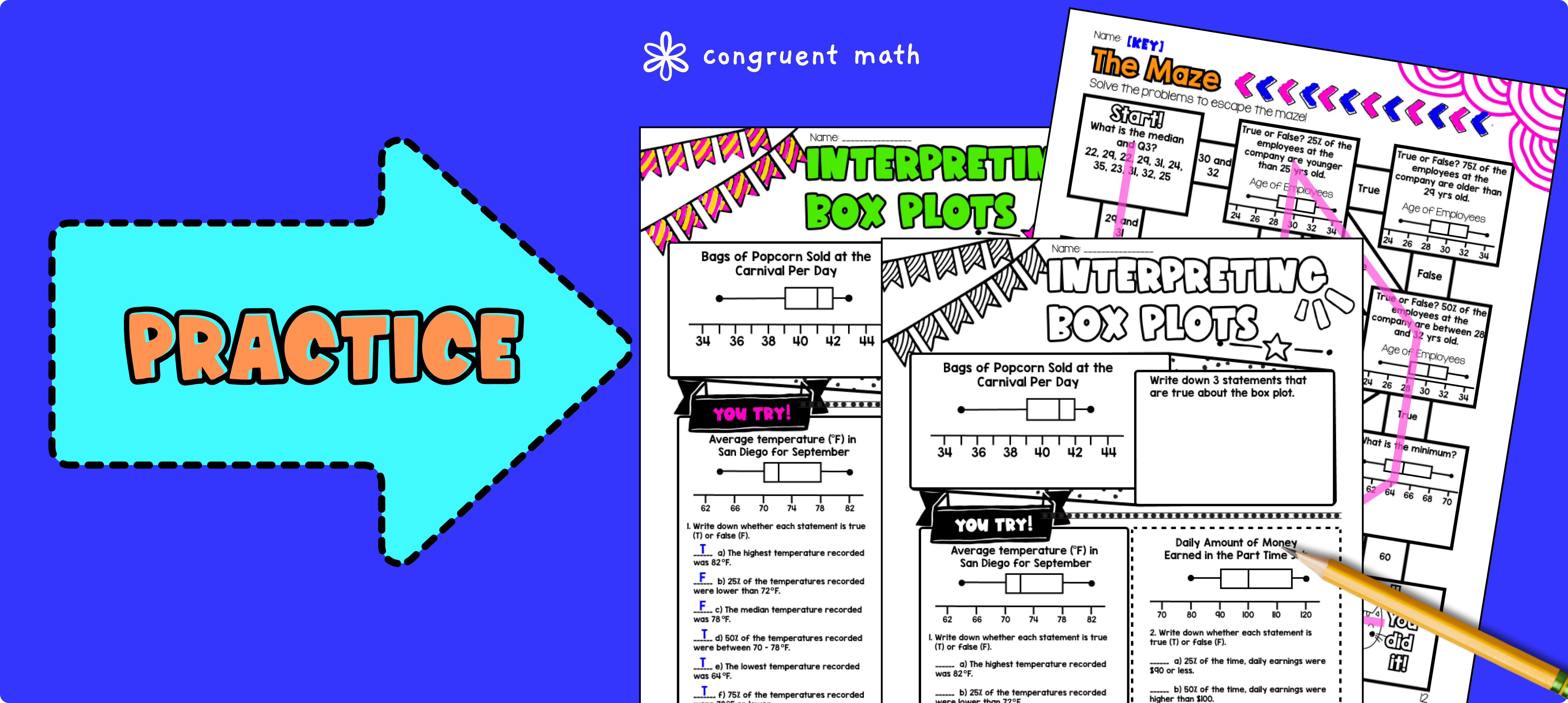Pin This

While students are working on the box and whisker plots practice sheet activity, walk around to answer student questions.

Fast finishers can dive into the Maze activity or doodle math (similar to color by number) for extra practice. You can assign it as homework for the remainder of the class.”

### Real-Life Application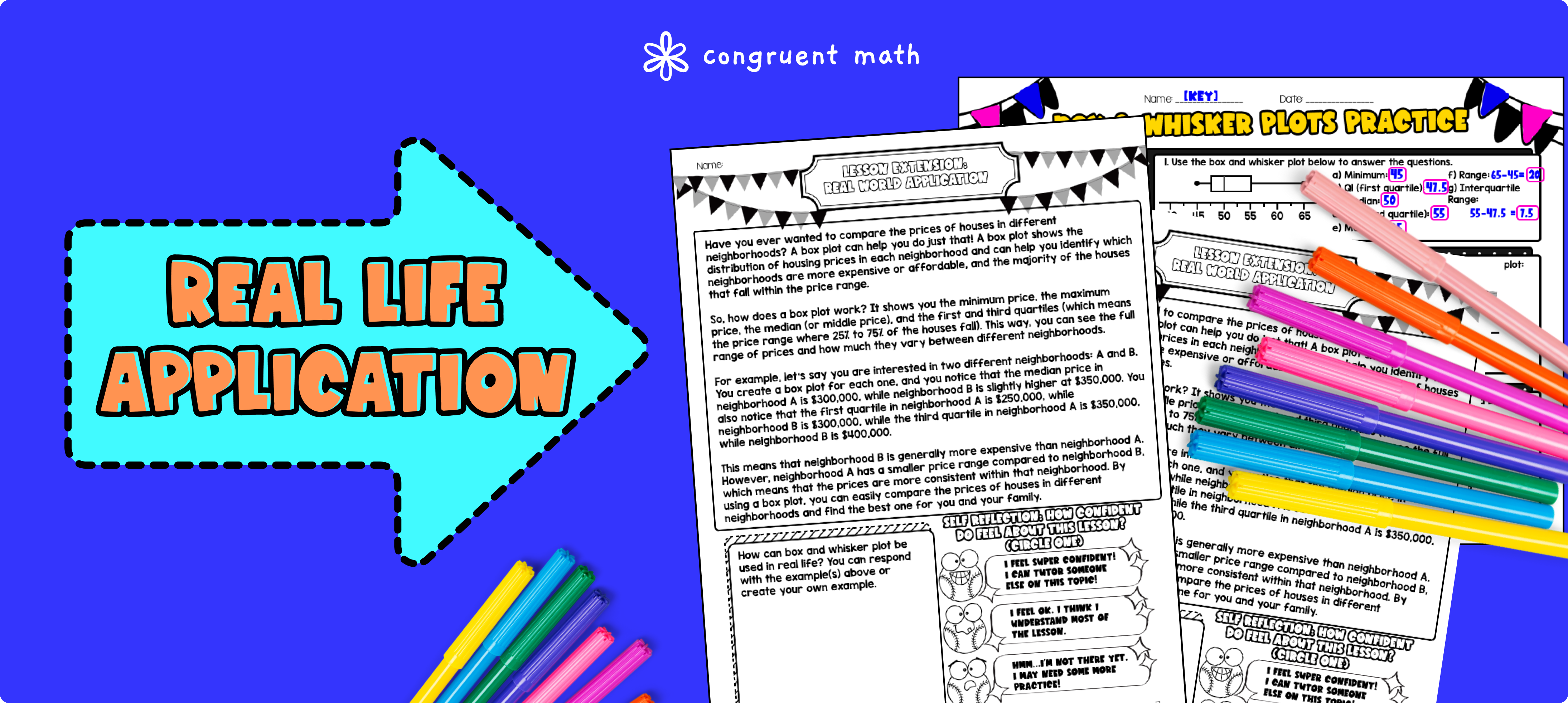Pin This

Bring the class back together, and introduce the concept of data visualization in comparing real estate. Explain how box plots can be used to compare the prices of houses in different neighborhoods. A box plot shows the distribution of housing prices in each neighborhood and can help you identify which neighborhoods are more expensive or affordable, and the majority of the houses that fall within the price range. Refer to the FAQ for more ideas on how to teach it!

## Extensions

If you're looking for digital practice for interpreting box and whisker plots, try the Pixel Art activity in Google Sheets. Every answer is automatically checked, and correct answers unlock parts of a mystery picture. It's incredibly fun, and a powerful tool for differentiation.